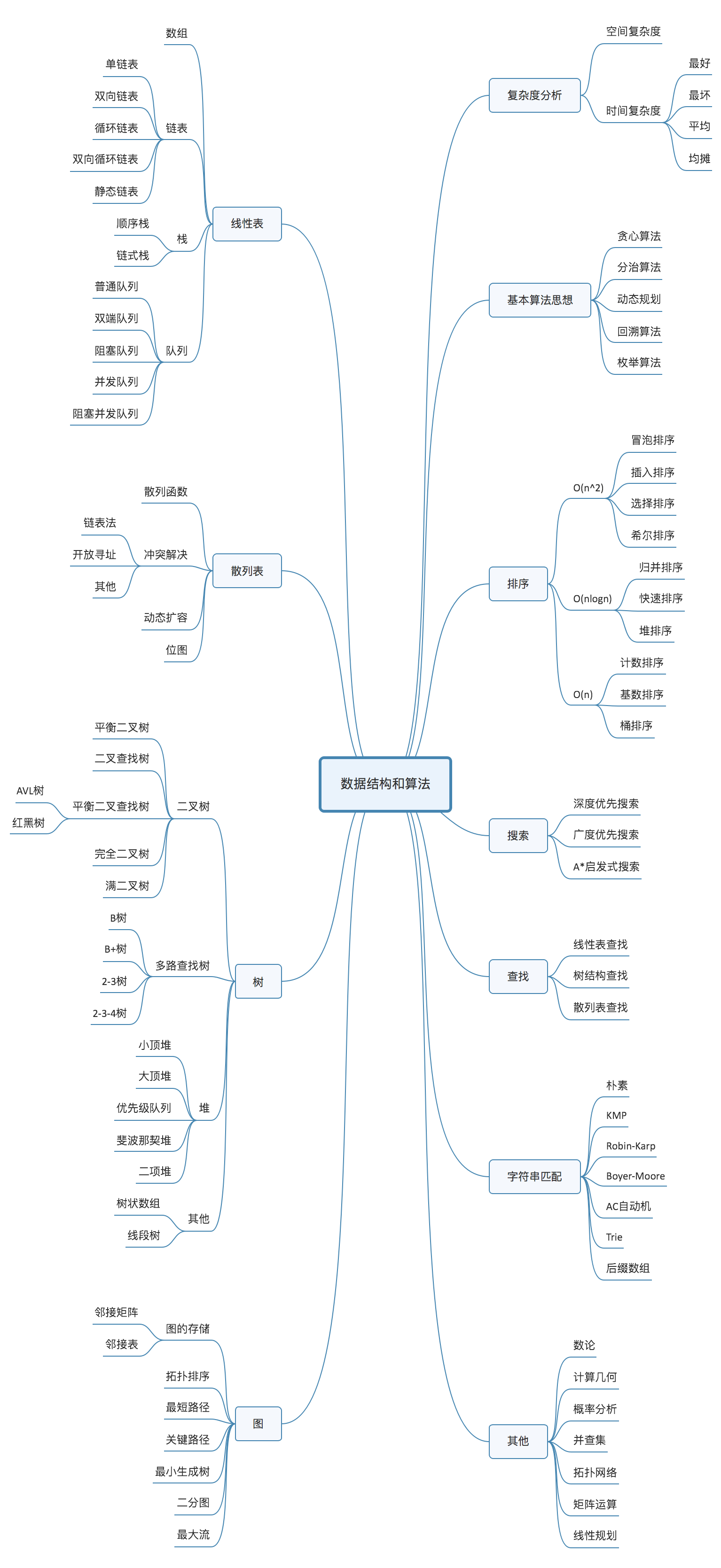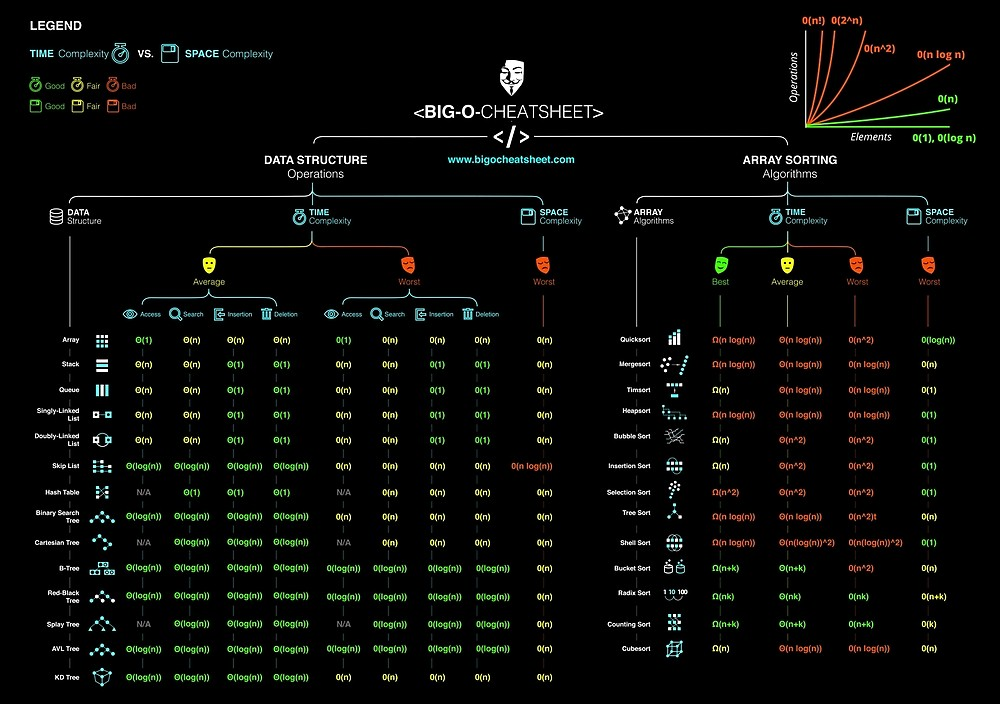# 《数据结构与算法之美 - 王争》¶## 复杂度分析¶### 分类¶

• 时间复杂度(asymptotic time complexity)

代码的执行时间随数据规模增长的变化趋势

• 空间复杂度(asymptotic space complexity)

代码的存储空间随数据规模增长的变化趋势

### 量级¶

• 多项式
• $O(1)$
• $O(n)$
• $O(\log(n))$
• $O(n\log(n))$
• $O(n^2)、O(n^3) ... O(n^k)$
• 非多项式
• $O(2^n)$
• $O(n!)$

### 情况¶

• 最好情况时间复杂度(best case time complexity)
• 最坏情况时间复杂度(worst case time complexity)
• 平均情况时间复杂度(average case time complexity)
• 均摊时间复杂度(amortized time complexity)

## 线性表(Linear List)¶

### 数组(Array)¶

• 随机访问和更新 O(1)
• 搜索、插入、删除 O(n)

• 单向链表、循环链表
• 双向链表（空间换时间）、双向循环链表

• 先进先出策略 FIFO(First In， First Out)
• 最少使用策略 LFU(Least Frequently Used)
• 最近最少使用策略 LRU(Least Recently Used)

## 递归(Recursion)¶

• 一个问题的解可以分解为几个子问题的解
• 这个问题与分解之后的子问题，除了数据规模不同，求解思路完全一样
• 存在终止条件